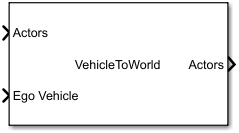Vehicle To World

Convert actors from ego vehicle coordinates to world coordinates

• Library:
• Automated Driving Toolbox / Driving Scenario and Sensor Modeling

•Description

The Vehicle To World block converts actor poses from the vehicle coordinates of the input ego vehicle to world coordinates. Use this block to convert non-ego actor poses output by the Scenario Reader block into world coordinates for use with the 3D simulation environment. Before using these output poses to specify vehicle positions in the 3D environment, first convert them from the cuboid to the 3D simulation world coordinate system by using a Cuboid To 3D Simulation block. For an example of this workflow, see the Visualize Sensor Data from Unreal Engine Simulation Environment example.

Ports

Input

expand all

Actor poses in vehicle coordinates, specified as a Simulink bus containing a MATLAB structure.

The structure must contain these fields.

FieldDescriptionType
NumActorsNumber of actorsNonnegative integer
TimeCurrent simulation timeReal-valued scalar
ActorsActor posesNumActors-length array of actor pose structures

Each actor pose structure in Actors must contain these fields.

FieldDescription
ActorID

Scenario-defined actor identifier, specified as a positive integer.

Position

Position of actor, specified as a real-valued vector of the form [x y z]. Units are in meters.

Velocity

Velocity (v) of actor in the x- y-, and z-directions, specified as a real-valued vector of the form [vx vy vz]. Units are in meters per second.

Roll

Roll angle of actor, specified as a real-valued scalar. Units are in degrees.

Pitch

Pitch angle of actor, specified as a real-valued scalar. Units are in degrees.

Yaw

Yaw angle of actor, specified as a real-valued scalar. Units are in degrees.

AngularVelocity

Angular velocity (ω) of actor in the x-, y-, and z-directions, specified as a real-valued vector of the form [ωx ωy ωz]. Units are in degrees per second.

Ego vehicle pose, specified as a Simulink bus containing a MATLAB structure.

The structure must contain these fields.

FieldDescription
ActorID

Scenario-defined actor identifier, specified as a positive integer.

Position

Position of actor, specified as a real-valued vector of the form [x y z]. Units are in meters.

Velocity

Velocity (v) of actor in the x- y-, and z-directions, specified as a real-valued vector of the form [vx vy vz]. Units are in meters per second.

Roll

Roll angle of actor, specified as a real-valued scalar. Units are in degrees.

Pitch

Pitch angle of actor, specified as a real-valued scalar. Units are in degrees.

Yaw

Yaw angle of actor, specified as a real-valued scalar. Units are in degrees.

AngularVelocity

Angular velocity (ω) of actor in the x-, y-, and z-directions, specified as a real-valued vector of the form [ωx ωy ωz]. Units are in degrees per second.

Output

expand all

Actor poses in world coordinates, returned as a Simulink bus containing a MATLAB structure.

The structure has these fields.

FieldDescriptionType
NumActorsNumber of actorsNonnegative integer
TimeCurrent simulation timeReal-valued scalar
ActorsActor posesNumActors-length array of actor pose structures

Each actor pose structure in Actors has these fields.

FieldDescription
ActorID

Scenario-defined actor identifier, specified as a positive integer.

Position

Position of actor, specified as a real-valued vector of the form [x y z]. Units are in meters.

Velocity

Velocity (v) of actor in the x- y-, and z-directions, specified as a real-valued vector of the form [vx vy vz]. Units are in meters per second.

Roll

Roll angle of actor, specified as a real-valued scalar. Units are in degrees.

Pitch

Pitch angle of actor, specified as a real-valued scalar. Units are in degrees.

Yaw

Yaw angle of actor, specified as a real-valued scalar. Units are in degrees.

AngularVelocity

Angular velocity (ω) of actor in the x-, y-, and z-directions, specified as a real-valued vector of the form [ωx ωy ωz]. Units are in degrees per second.

Parameters

expand all

Source of the name for the actor poses bus returned in the Actors output port, specified as one of these options:

• Auto — The block automatically creates an actor poses bus name.

• Property — Specify the actor poses bus name by using the Actors bus name parameter.

Name of the actor poses bus returned in the Actors output port, specified as a valid bus name.

Dependencies

To enable this parameter, set Source of actors bus name to Property.

• Interpreted execution — Simulate the model using the MATLAB interpreter. This option shortens startup time. In Interpreted execution mode, you can debug the source code of the block.

• Code generation — Simulate the model using generated C/C++ code. The first time you run a simulation, Simulink generates C/C++ code for the block. The C code is reused for subsequent simulations as long as the model does not change. This option requires additional startup time.

Extended Capabilities

C/C++ Code GenerationGenerate C and C++ code using Simulink® Coder™.

Introduced in R2020a